We're building the future of Supply Chain Security. View Openings

## Risk Ledger Blog

The latest updates, stories, ideas and guides from the Risk Ledger team.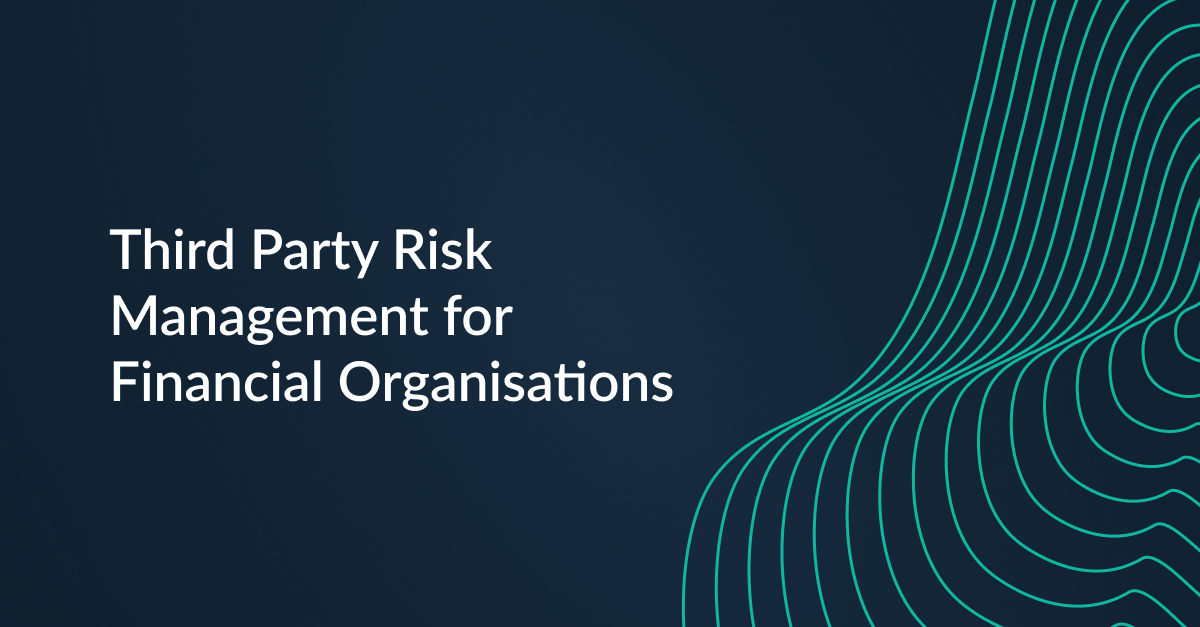Risk LedgerRisk LedgerRisk LedgerRisk Ledger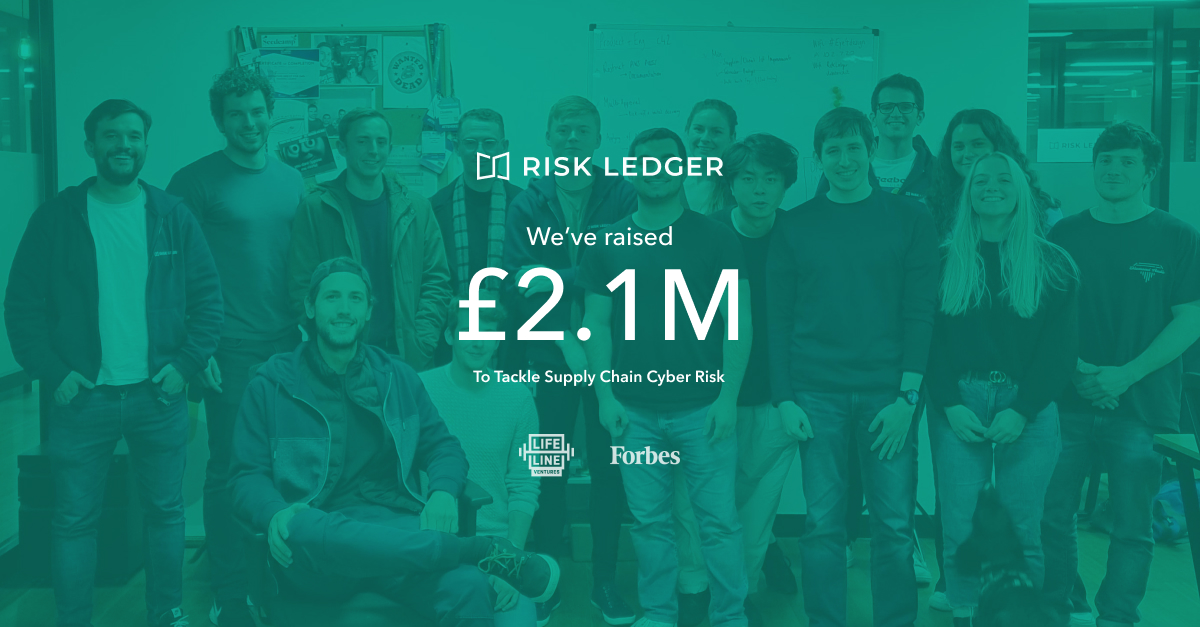Risk LedgerRisk Ledger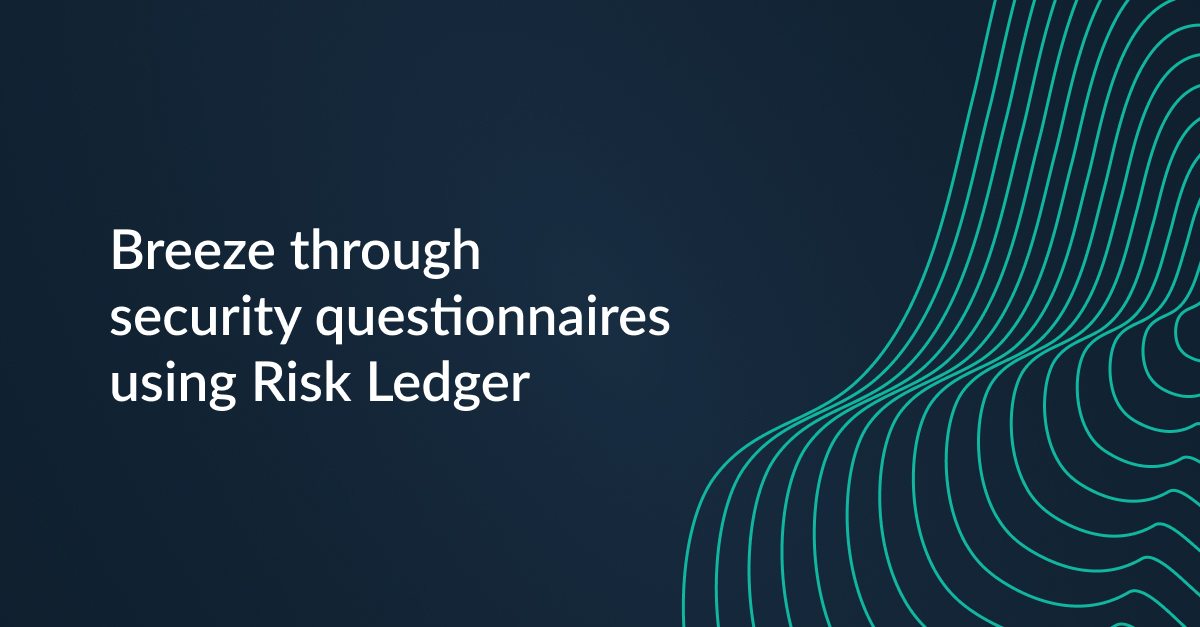Laura WhiteLaura White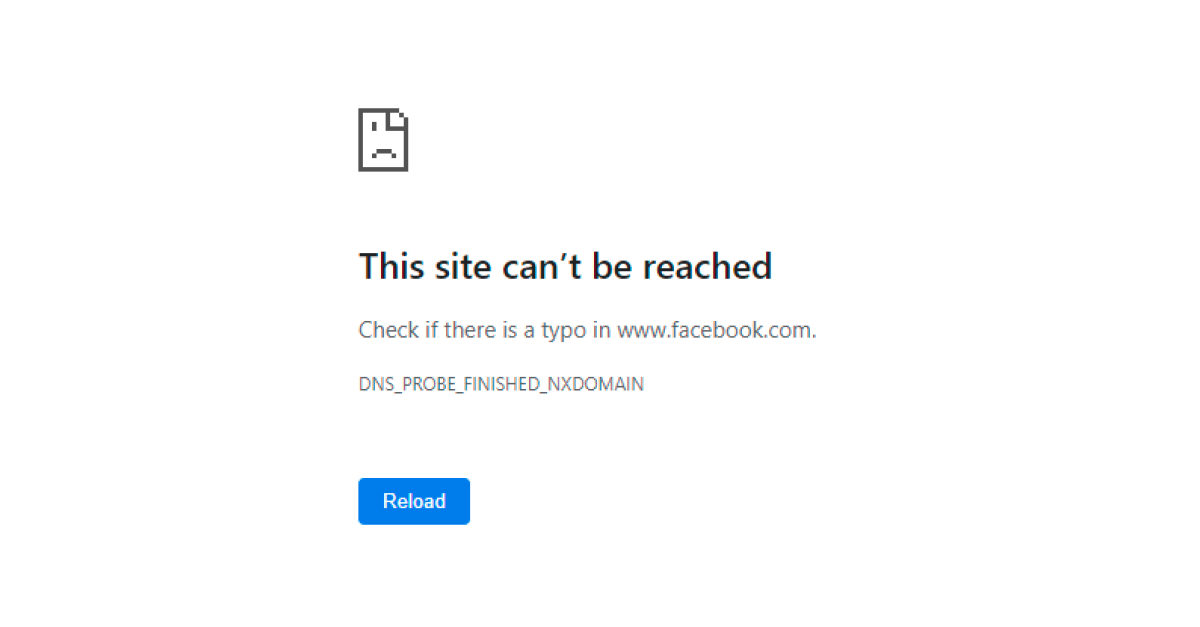James CunninghamJames Cunningham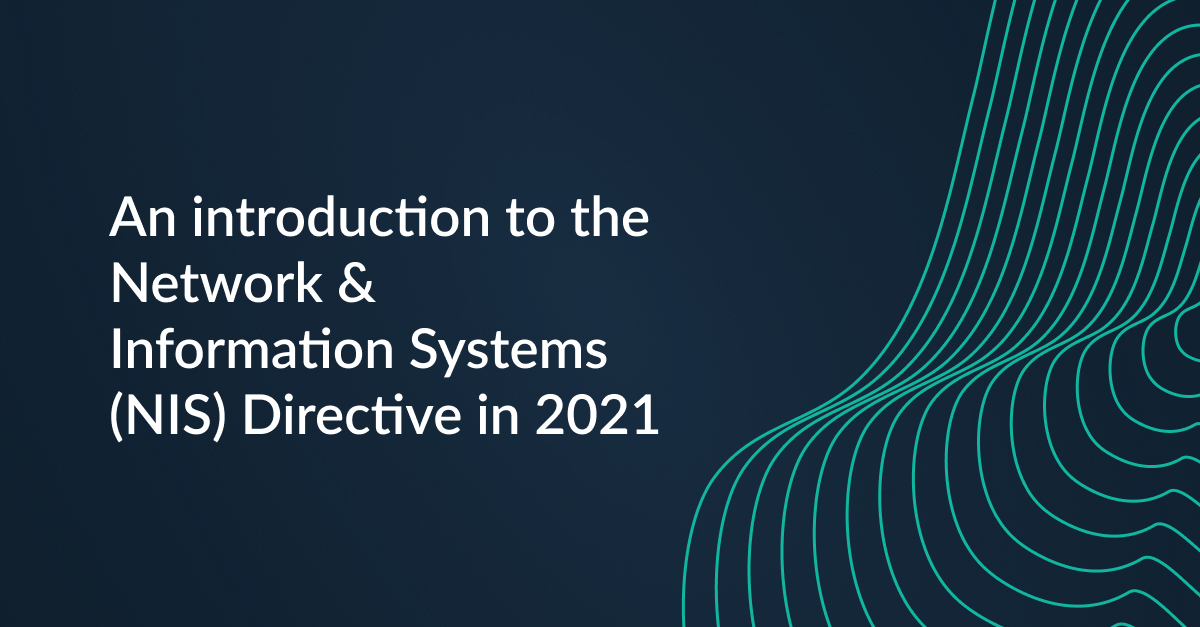Risk LedgerRisk Ledger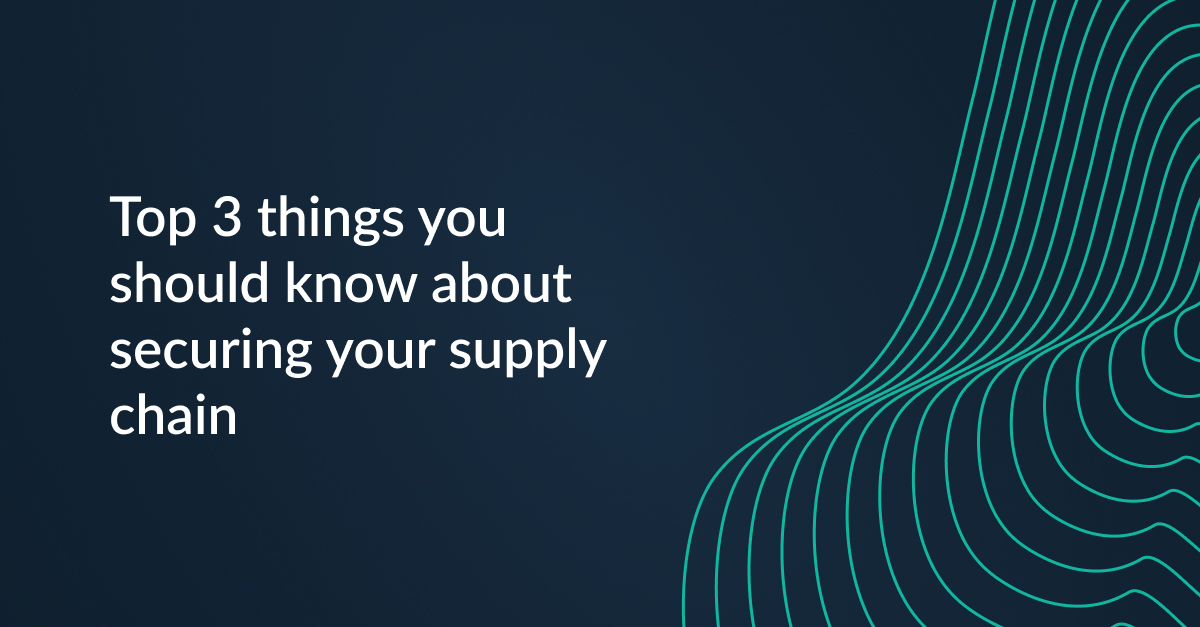Risk LedgerRisk Ledger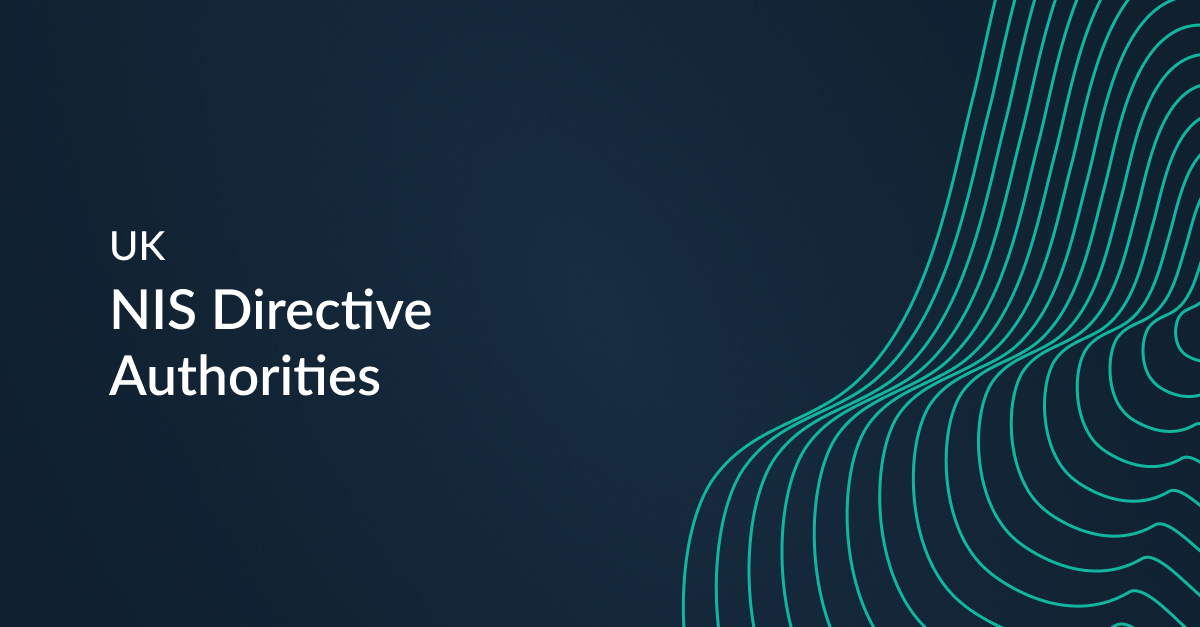Risk LedgerRisk LedgerBruno CalogeroBruno Calogero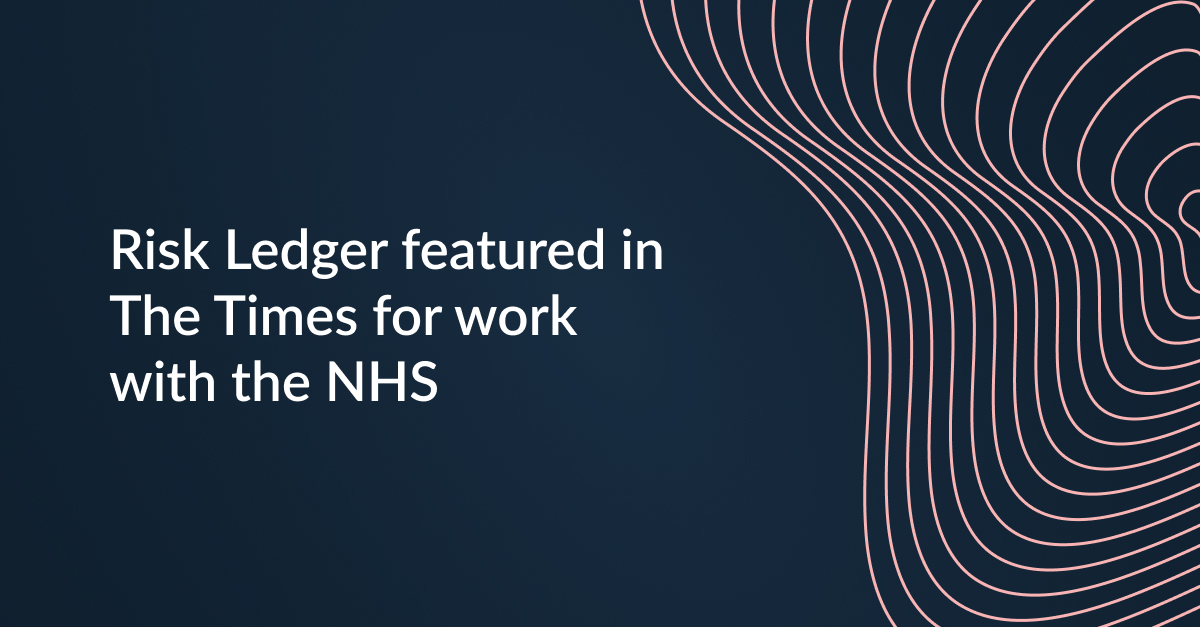Risk LedgerRisk Ledger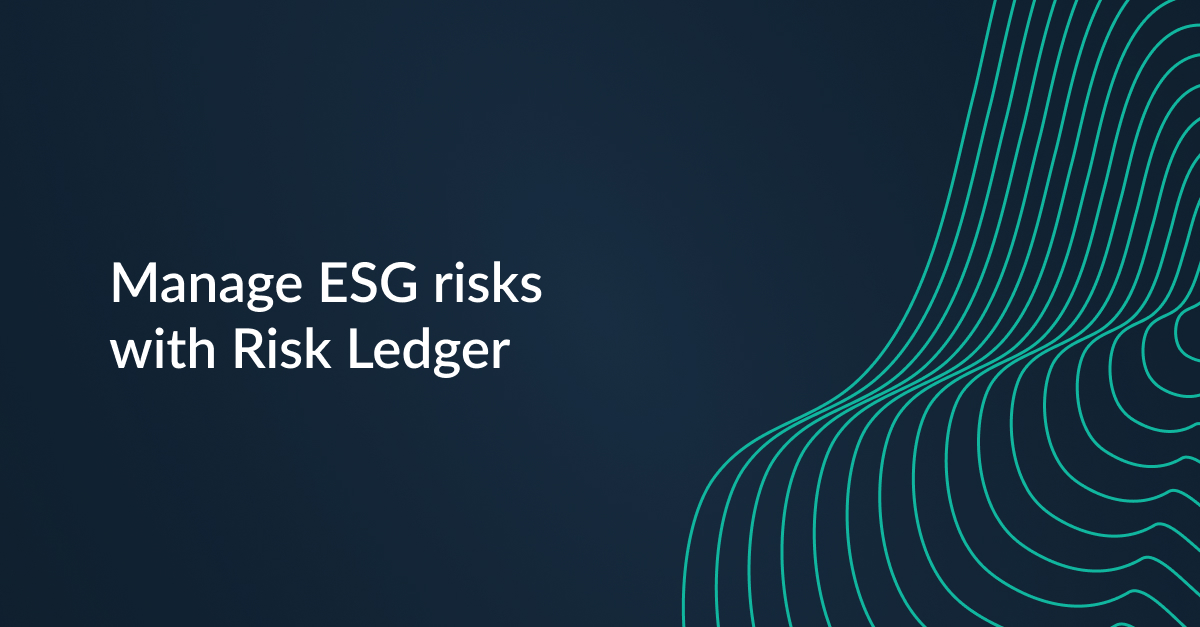Risk LedgerRisk Ledger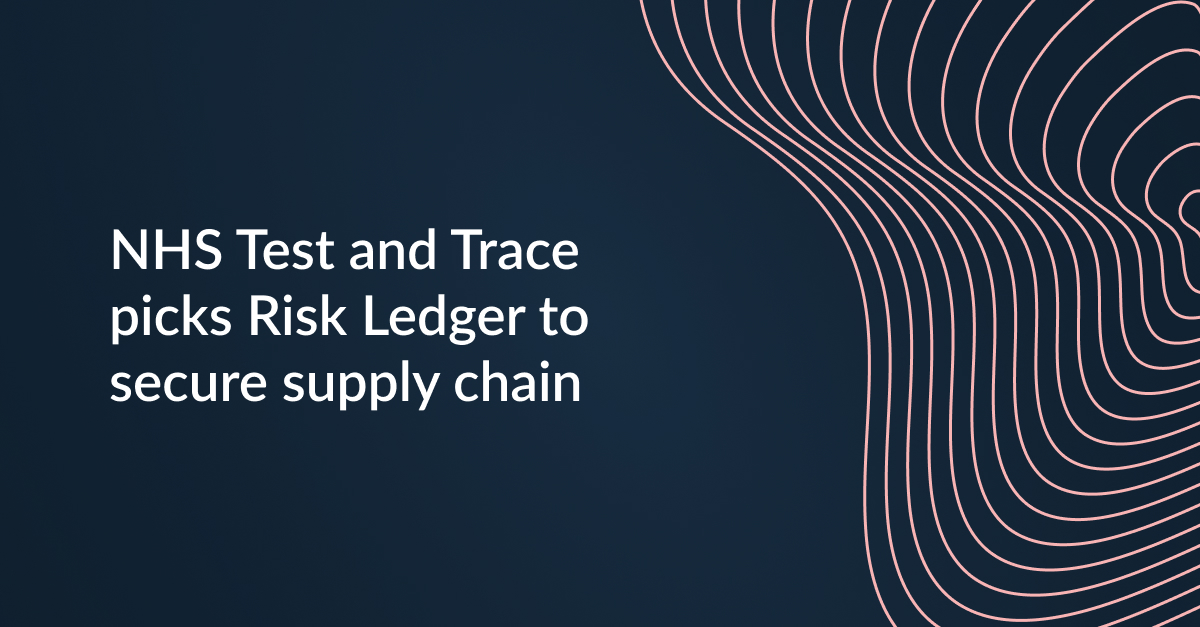Risk LedgerRisk Ledger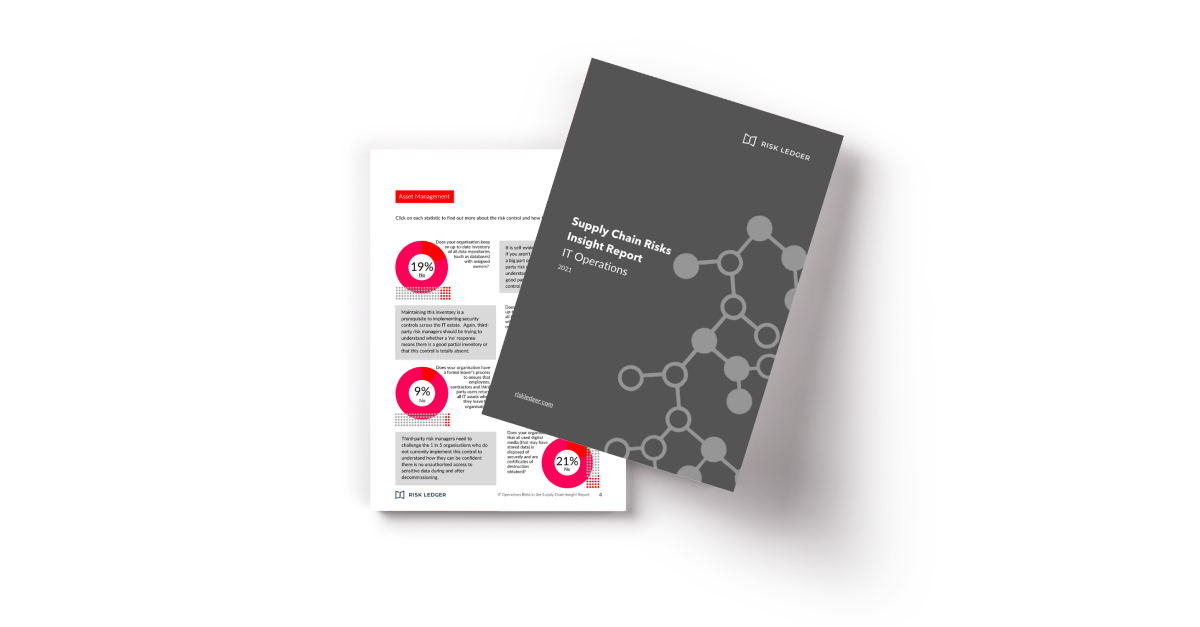Risk LedgerRisk LedgerRisk LedgerRisk Ledger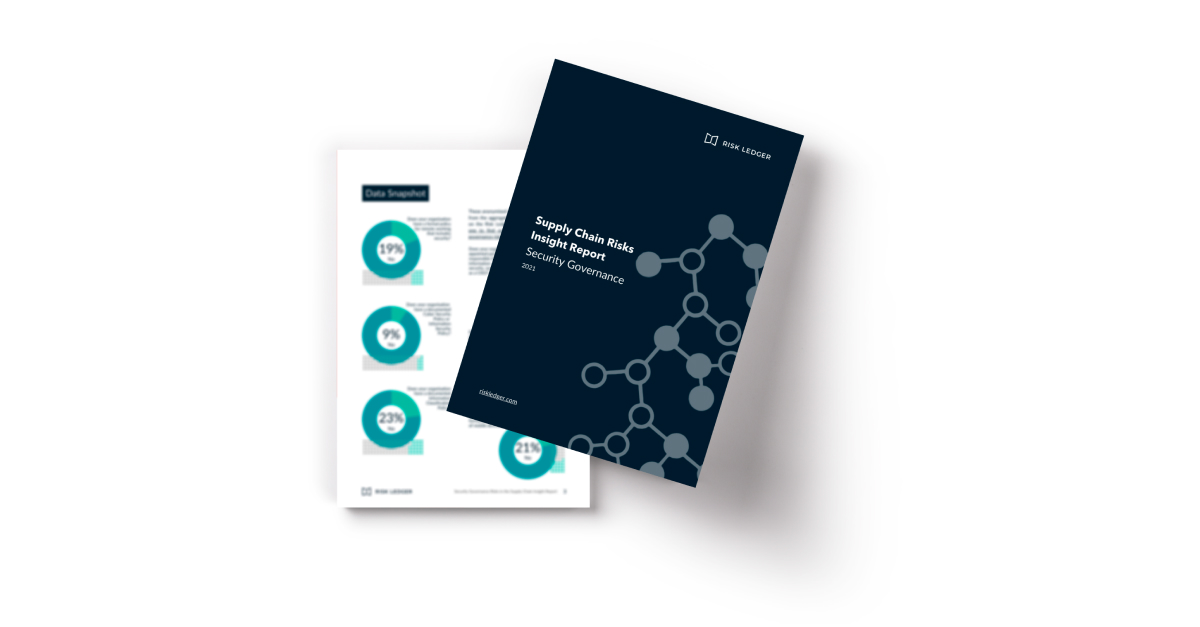Risk LedgerRisk Ledger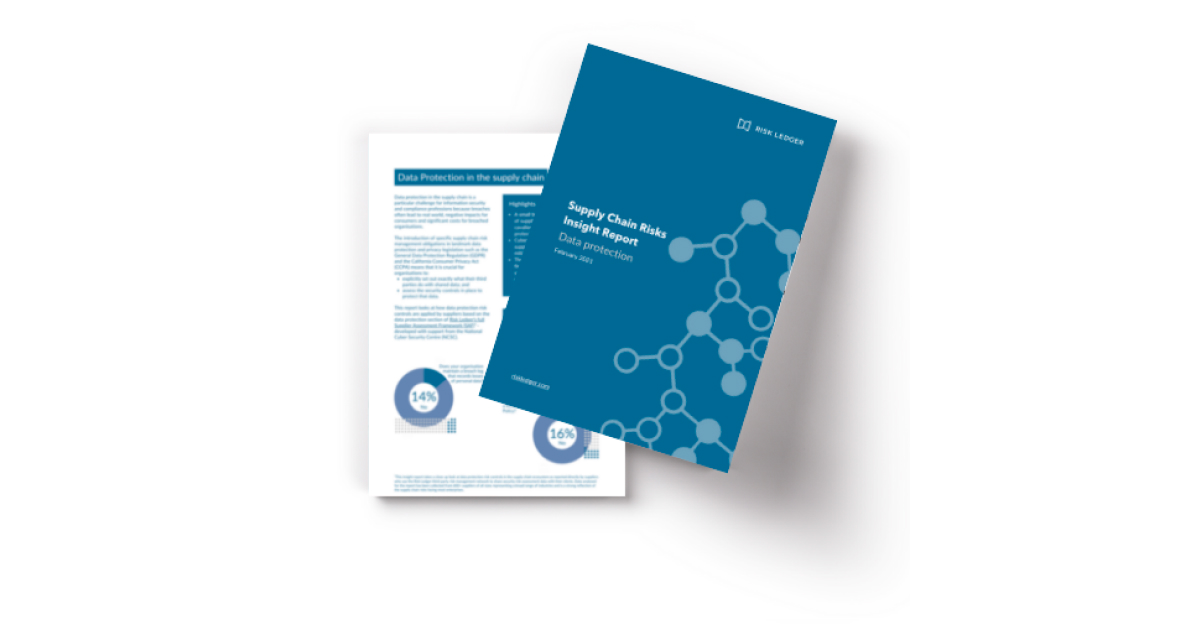Risk LedgerRisk LedgerRisk LedgerRisk Ledger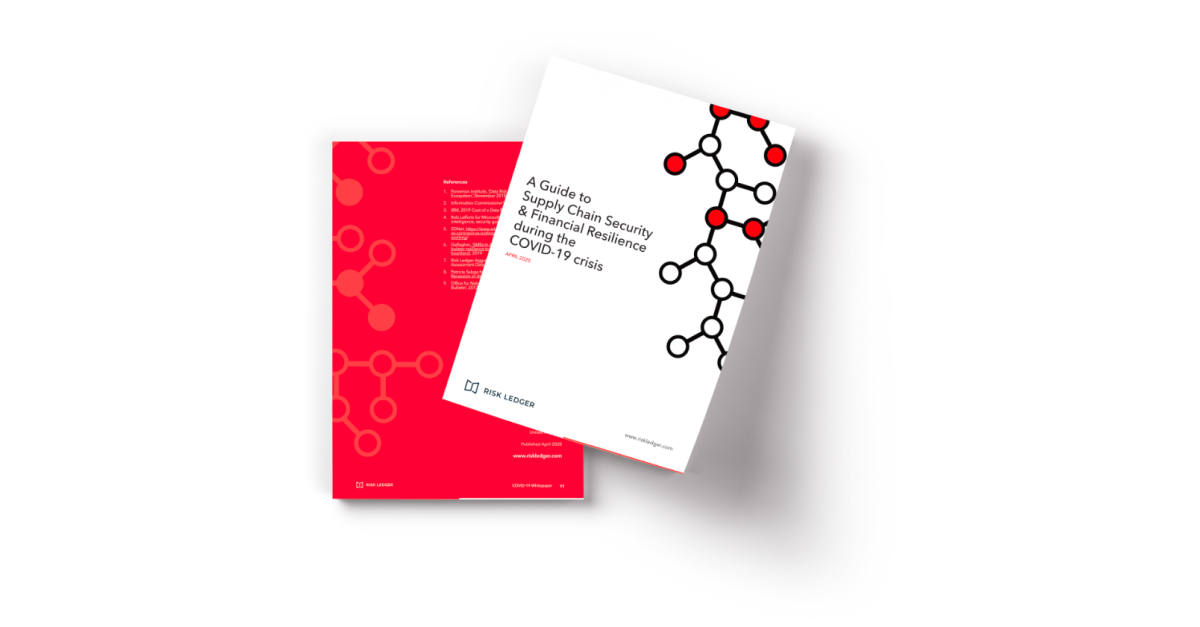Risk LedgerRisk Ledger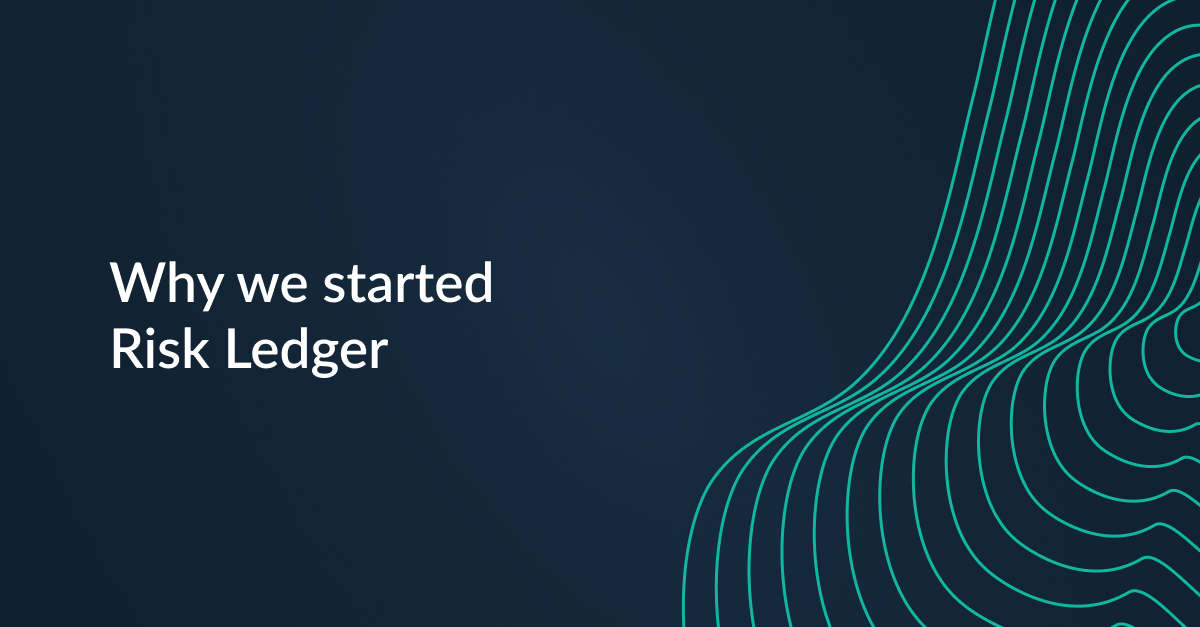Risk LedgerRisk Ledger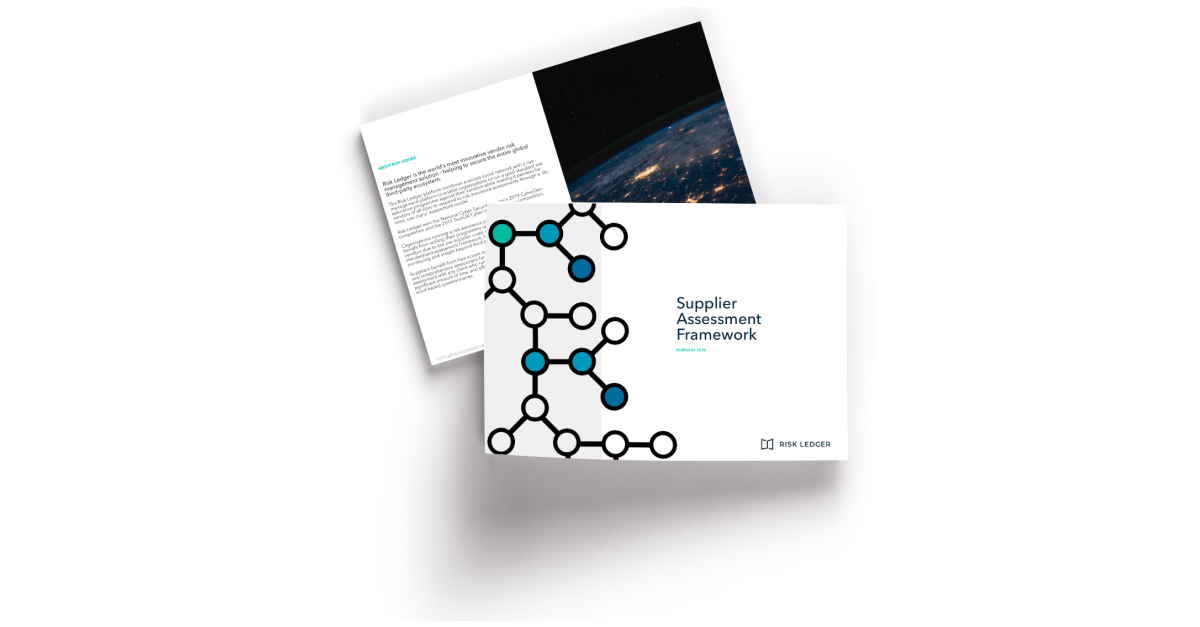Risk LedgerRisk LedgerRisk LedgerRisk LedgerRisk LedgerDan & Haydn